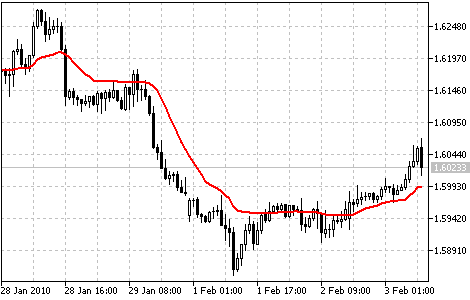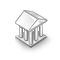Interesting script?
So post a link to it -
let others appraise it
You liked the script? Try it in the MetaTrader 5 terminal# Variable Index Dynamic Average (VIDYA) - indicator for MetaTrader 5

Views:
12294
Rating:
Published:
2010.02.03 14:04
Updated:
2016.11.22 07:32

Variable Index Dynamic Average (VIDYA) technical indicator was developed by Tushar Chande.

It is an original method of calculating the Exponential Moving Average (EMA) with the dynamically changing period of averaging. Period of averaging depends on the market volatility; as the measure of volatility Chande Momentum Oscillator (CMO) was chosen.

This oscillator measures the ratio between the sum of positive increments and sum of negative increments for a certain period (CMO period). CMO value is used as the ratio to the smoothing factor EMA. Thus VIDYA has to setup parameters: period of CMO and period of EMA.

Application

Usually not VIDYA itself is used in trading systems, but its upper and lower borders (Upper band & Lower band), that are by N% above and below VIDYA. Interpretation of the indicator for receiving trade signals in this form is performed similar to Bollinger Bands ®.Variable Index Dynamic Average Indicator

Calculation:

The standard Exponential Moving Average is calculated according to the below formula:

EMA(i) = Price(i) * F + EMA(i-1)*(1-F)

where:

• F = 2/(Period_EMA+1) - smoothing factor;
• Period_EMA - EMA averaging period;
• Price(i) - current price;
• EMA(i-1) - previous value of EMA.

The value of Variable Index Dynamic Average is calculated in the analogous way using CMO:

VIDYA(i) = Price(i) * F * ABS(CMO(i)) + VIDYA(i-1) * (1 - F* ABS(CMO(i)))

where:

• ABS(CMO(i)) - absolute current value Chande Momentum Oscillator;
• VIDYA(i-1) - previous value of VIDYA.

The value of CMO is calculated according to the below formula:

CMO(i) = (UpSum(i) - DnSum(i))/(UpSum(i) + DnSum(i))

where:

• UpSum(i) - current sum of positive price increments for the period;
• DnSum(i) - current sum of negative price increments for the period.Triple Exponential Moving Average (TEMA)

TEMA can be used instead of traditional moving averages. It can be used for smoothing price data, as well as for smoothing other indicators.Double Exponential Moving Average (DEMA)

It is used for smoothing price series and is applied directly on a price chart of a financial security.Triple Exponential Average (TRIX)

It's an oscillator of the overbought/oversold market conditions. It can also be used as the Momentum indicator. Triple smoothing is used for removing the cyclic components in price movements with the period less than that of TRIX.MovingAverages

The MovingAverages library contains functions for calculation of different types of moving averages.